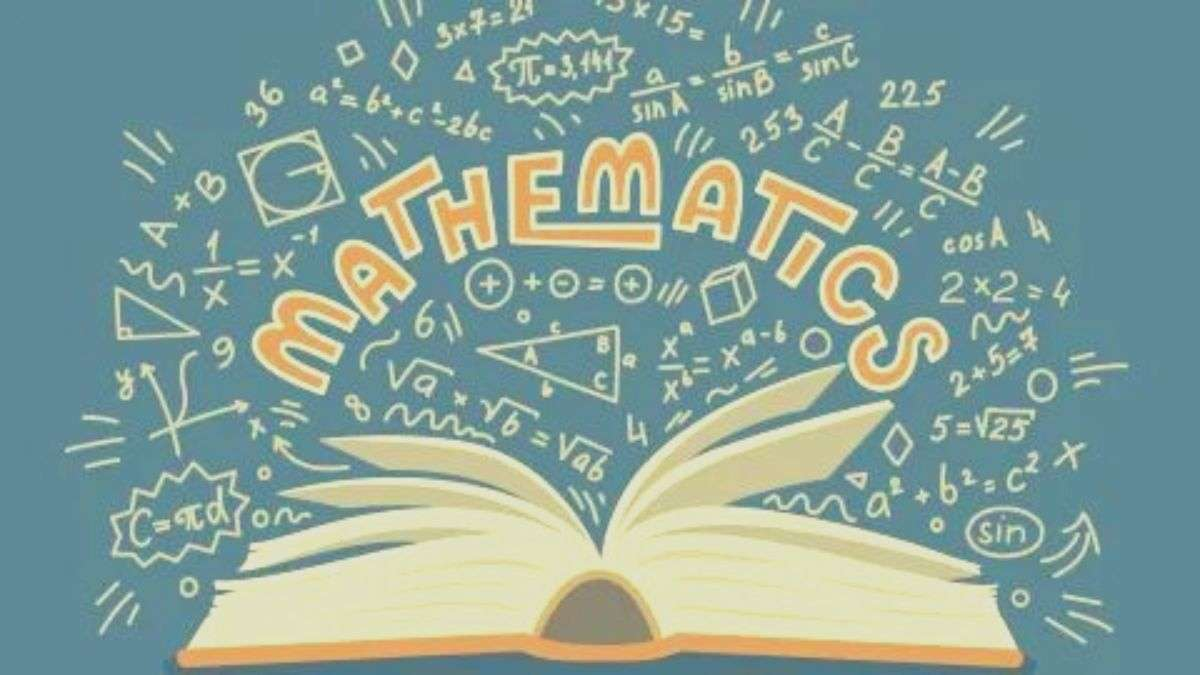# Math riddles with answers: Math is definitely not a boring subject. These math riddles are proof!

Every now and then we bring forward some exciting challenges and math riddles for you and today is no exception. Solve these exciting math riddles if you can.Long years ago, people invented, or rather, discovered the subject of mathematics with the thought that the discipline had the power to change the world. And yes, they were right in the approach. Math has solved trillions of human problems and continues to do so till now. Just like language, math too starts with humankind, is ever-evolving, and will never die. However, little did the discoverers know that the super interesting subject they discovered would actually become the most hated subject of students. Yes, whether you talk to a group of toppers who do not score less than 99 percent in school, or whether you talk to a group of students who wrote their destiny outside of textbooks, most of them frown at the very thought of mathematics. Life is full of problems and tribulations, and what reaction would anyone expect out of a subject that is completely filled with problems? However, the perception through which students look at the subject of mathematics is actually sorrowful.

Hey, let us bring some exciting math riddles for you that will surely change your perception of the subject!

### Math Riddle 1:

Sam is an expert at calculations. He is never wrong with mathematics. He added six to eleven, and the answer he gets is five. Is he correct with his calculations this time?

### Math Riddle 2:

What three numbers give the same answer whether they are multiplied or they are added?

### Math Riddle 3:

Name the smallest whole number that is actually equal to seven times the sum of its digits.

Math Riddle 1:

Sam is an expert at calculations. He is never wrong with mathematics. He added six to eleven, and the answer he gets is five. Is he correct with his calculations this time?

Yes, Sam is correct because he added six to eleven on the clock, and gets 5 as the resultant time.

Math Riddle 2:
What three numbers give the same answer whether they are multiplied or they are added?

The three numbers are 1, 2, and 3. Multiply or add them and you will get 6 as the answer.

Math Riddle 3:
Name the smallest whole number that is actually equal to seven times the sum of its digits.

The smallest whole number here is 21.

Weren’t these math riddles interesting?

Optical Illusion: Finding the hidden parrot is super easy and super fun! Try this fun challenge!

Get the latest General Knowledge and Current Affairs from all over India and world for all competitive exams.
खेलें हर किस्म के रोमांच से भरपूर गेम्स सिर्फ़ जागरण प्ले पर